/

### Integration of Piecewise Polynomials against a Gaussian probability density function

In a number of financial contexts it can be important to calculate the following integral: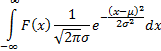For example, this integral is relevant to calculating moments of a fat-tailed distribution, i.e. one whose quantile-quantile plot versus the Normal distribution,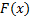, is not unity. The faster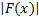increases as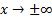the greater is the impact of fat-tailed behaviour, i.e. deviations from a density function of the form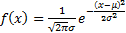. Thus risk measures such as expected shortfall (effectively a first moment computation, in which the leading element ofis of order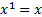) are more sensitive to fat-tailed effects than Value-at-Risk risk measures (effectively a zero moment computation, in which the leading element ofis of order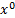).

This integral can also appear in derivative pricing analysis, if payoff is being approximated by a piecewise polynomial function and the movement of the underlying is of a certain type (but see Valuing polynomial payoffs in a Black Scholes World, which suggests that some modification may be needed to cater for exponentials arising when converting the partial differential equation arising under a Gauss-Weiner process to one with ‘standard’ parabolic form).

Hence, it becomes helpful to be able to calculate the following integral rapidly: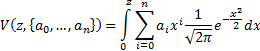Ifis large then we note that: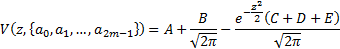Where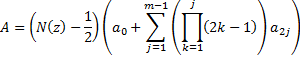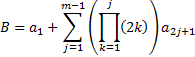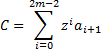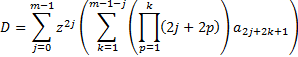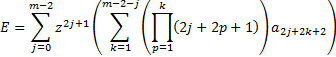Ifis small then for higher order coefficients the above computation runs into machine rounding problems. It is then better to use a Taylor series expansion, bearing in mind that: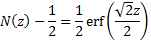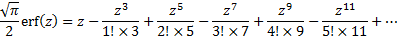Hence (ifis integral and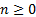) we have: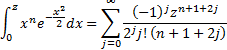Software Research Consulting Calculator Publications Examples Functions ERM Education Spreadsheets References Contact us HTML/CSS/JS Other

© 2021 - Nematrian Limited

This site uses cookies to improve and monitor its performance. Cookies may already be placed on your computer. By continuing to use this site, you are agreeing to accept these cookies which can be deleted at any time.
License Agreement etc. | Disclaimer
Desktop view | Switch to Mobile